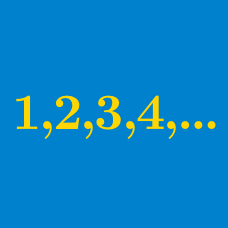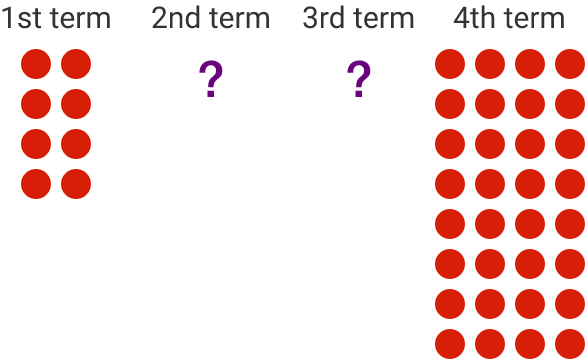Algebra

# Finding the Common Difference - Arithmetic Progressions

Given the arithmetic progression

$10 ,7, 4, 1, x,$

what is $$x$$?

My doctor gave me some pills with the following instructions:

"Take 12 pills on the first day, and then reduce the amount by 3 pills on each subsequent day until you don't have to take any."

When will be the first day that I don't have to take any pills?

Which of the following sequences is NOT an arithmetic progression?

(A) $$\{ 11, 5, -1, -7, -13, \cdots \}$$
(B) $$\{ 5, 5, 5, 5, 5, \cdots \}$$
(C) $$\{ 1, 2, 4, 6, 8, \cdots \}$$The numbers of red circles in each group in the above diagram form an arithmetic progression. What is the common difference?

$2, a, 5, b, 8$

If the above numbers form an arithmetic progression, what is its common difference?

×